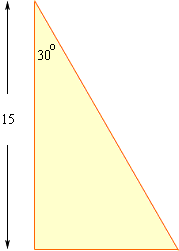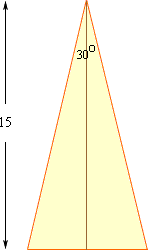Quandaries and Queries With a 30 degree angle at the top of a triangle and a height of 15 what is the base? Ralph Hi Ralph, You can have triangles with the angle at the top being 30 degrees and the height being 15 units and yet the bases are of different lengths. For example if the triangle is a right trianglethen the length of the base is 15/root(3) which is approximately 8.66 units. If the triangle is an isosceles triangle with height 15 units and apex angle of 30 degreesthen the length of the base is 215tan(15o) which is approximately 8.04 units. Penny Go to Math Central